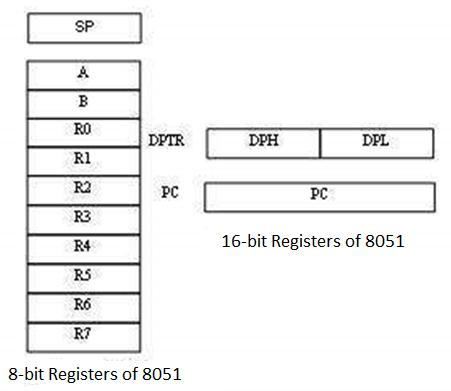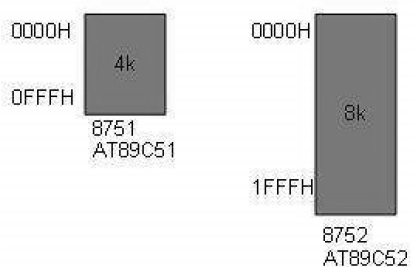# Embedded Systems - Registers

Registers are used in the CPU to store information on temporarily basis which could be data to be processed, or an address pointing to the data which is to be fetched. In 8051, there is one data type is of 8-bits, from the MSB (most significant bit) D7 to the LSB (least significant bit) D0. With 8-bit data type, any data type larger than 8-bits must be broken into 8-bit chunks before it is processed.

The most widely used registers of the 8051 are A (accumulator), B, R0-R7, DPTR (data pointer), and PC (program counter). All these registers are of 8-bits, except DPTR and PC.

## Storage Registers in 8051

We will discuss the following types of storage registers here −

• Accumulator
• R register
• B register
• Data Pointer (DPTR)
• Program Counter (PC)
• Stack Pointer (SP)

### Accumulator

The accumulator, register A, is used for all arithmetic and logic operations. If the accumulator is not present, then every result of each calculation (addition, multiplication, shift, etc.) is to be stored into the main memory. Access to main memory is slower than access to a register like the accumulator because the technology used for the large main memory is slower (but cheaper) than that used for a register.

### The "R" Registers

The "R" registers are a set of eight registers, namely, R0, R1 to R7. These registers function as auxiliary or temporary storage registers in many operations. Consider an example of the sum of 10 and 20. Store a variable 10 in an accumulator and another variable 20 in, say, register R4. To process the addition operation, execute the following command −

```ADD A,R4
```

After executing this instruction, the accumulator will contain the value 30. Thus "R" registers are very important auxiliary or helper registers. The Accumulator alone would not be very useful if it were not for these "R" registers. The "R" registers are meant for temporarily storage of values.

Let us take another example. We will add the values in R1 and R2 together and then subtract the values of R3 and R4 from the result.

```MOV A,R3   ;Move the value of R3 into the accumulator
MOV R5,A   ;Store the resulting value temporarily in R5
MOV A,R1   ;Move the value of R1 into the accumulator
SUBB A,R5  ;Subtract the value of R5 (which now contains R3 + R4)
```

As you can see, we used R5 to temporarily hold the sum of R3 and R4. Of course, this is not the most efficient way to calculate (R1 + R2) – (R3 + R4), but it does illustrate the use of the "R" registers as a way to store values temporarily.### The "B" Register

The "B" register is very similar to the Accumulator in the sense that it may hold an 8-bit (1-byte) value. The "B" register is used only by two 8051 instructions: MUL AB and DIV AB. To quickly and easily multiply or divide A by another number, you may store the other number in "B" and make use of these two instructions. Apart from using MUL and DIV instructions, the "B" register is often used as yet another temporary storage register, much like a ninth R register.

### The Data Pointer

The Data Pointer (DPTR) is the 8051’s only user-accessible 16-bit (2-byte) register. The Accumulator, R0–R7 registers and B register are 1-byte value registers. DPTR is meant for pointing to data. It is used by the 8051 to access external memory using the address indicated by DPTR. DPTR is the only 16-bit register available and is often used to store 2-byte values.

### The Program Counter

The Program Counter (PC) is a 2-byte address which tells the 8051 where the next instruction to execute can be found in the memory. PC starts at 0000h when the 8051 initializes and is incremented every time after an instruction is executed. PC is not always incremented by 1. Some instructions may require 2 or 3 bytes; in such cases, the PC will be incremented by 2 or 3.

Branch, jump, and interrupt operations load the Program Counter with an address other than the next sequential location. Activating a power-on reset will cause all values in the register to be lost. It means the value of the PC is 0 upon reset, forcing the CPU to fetch the first opcode from the ROM location 0000. It means we must place the first byte of upcode in ROM location 0000 because that is where the CPU expects to find the first instruction.

### The Stack Pointer (SP)

The Stack Pointer, like all registers except DPTR and PC, may hold an 8-bit (1-byte) value. The Stack Pointer tells the location from where the next value is to be removed from the stack. When a value is pushed onto the stack, the value of SP is incremented and then the value is stored at the resulting memory location. When a value is popped off the stack, the value is returned from the memory location indicated by SP, and then the value of SP is decremented.

This order of operation is important. SP will be initialized to 07h when the 8051 is initialized. If a value is pushed onto the stack at the same time, the value will be stored in the internal RAM address 08h because the 8051 will first increment the value of SP (from 07h to 08h) and then will store the pushed value at that memory address (08h). SP is modified directly by the 8051 by six instructions: PUSH, POP, ACALL, LCALL, RET, and RETI.

## ROM Space in 8051

Some family members of 8051 have only 4K bytes of on-chip ROM (e.g. 8751, AT8951); some have 8K ROM like AT89C52, and there are some family members with 32K bytes and 64K bytes of on-chip ROM such as Dallas Semiconductor. The point to remember is that no member of the 8051 family can access more than 64K bytes of opcode since the program counter in 8051 is a 16-bit register (0000 to FFFF address).

The first location of the program ROM inside the 8051 has the address of 0000H, whereas the last location can be different depending on the size of the ROM on the chip. Among the 8051 family members, AT8951 has \$k bytes of on-chip ROM having a memory address of 0000 (first location) to 0FFFH (last location).### 8051 Flag Bits and PSW Register

The program status word (PSW) register is an 8-bit register, also known as flag register. It is of 8-bit wide but only 6-bit of it is used. The two unused bits are user-defined flags. Four of the flags are called conditional flags, which means that they indicate a condition which results after an instruction is executed. These four are CY (Carry), AC (auxiliary carry), P (parity), and OV (overflow). The bits RS0 and RS1 are used to change the bank registers. The following figure shows the program status word register.

The PSW Register contains that status bits that reflect the current status of the CPU.

CY CA F0 RS1 RS0 OV - P

 CY PSW.7 Carry Flag AC PSW.6 Auxiliary Carry Flag F0 PSW.5 Flag 0 available to user for general purpose. RS1 PSW.4 Register Bank selector bit 1 RS0 PSW.3 Register Bank selector bit 0 OV PSW.2 Overflow Flag - PSW.1 User definable FLAG P PSW.0 Parity FLAG. Set/ cleared by hardware during instruction cycle to indicate even/odd number of 1 bit in accumulator.

We can select the corresponding Register Bank bit using RS0 and RS1 bits.

0 0 0 00H-07H
0 1 1 08H-0FH
1 0 2 10H-17H
1 1 3 18H-1FH
• CY, the carry flag − This carry flag is set (1) whenever there is a carry out from the D7 bit. It is affected after an 8-bit addition or subtraction operation. It can also be reset to 1 or 0 directly by an instruction such as "SETB C" and "CLR C" where "SETB" stands for set bit carry and "CLR" stands for clear carry.

• AC, auxiliary carry flag − If there is a carry from D3 and D4 during an ADD or SUB operation, the AC bit is set; otherwise, it is cleared. It is used for the instruction to perform binary coded decimal arithmetic.

• P, the parity flag − The parity flag represents the number of 1's in the accumulator register only. If the A register contains odd number of 1's, then P = 1; and for even number of 1's, P = 0.

• OV, the overflow flag − This flag is set whenever the result of a signed number operation is too large causing the high-order bit to overflow into the sign bit. It is used only to detect errors in signed arithmetic operations.

### Example

Show the status of CY, AC, and P flags after the addition of 9CH and 64H in the following instruction.

MOV A, #9CH

```Solution:  9C   10011100